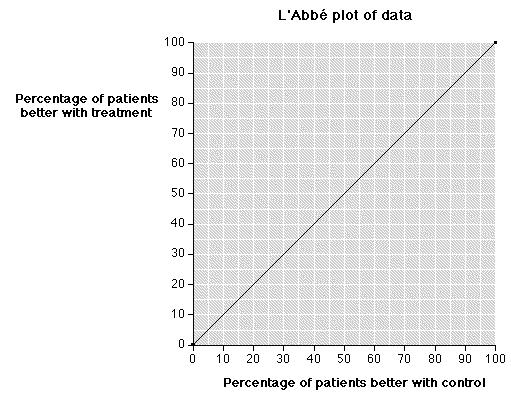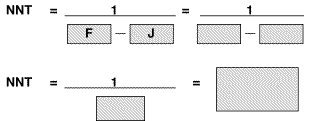# Numbers Needed to Treat (NNT) calculator

A number needed to treat (NNT) is defined by a number of characteristics. This worksheet is designed as an aide memoir for working out NNTs from papers and systematic reviews. First fill in the answers to the questions, where appropriate, graph the data on the LAbbé plot, and finally do the NNT calculation.

 Question/Action Answer A What is the intervention (ie drug dose & frequency)? B What is the intervention for? C What is the successful outcome (and when or over what time did it occur)? D How many had the intervention? E How many had a successful outcome with the intervention? F Express this as a percentage (100 x E/D) and as a proportion (E/D) G What is the control or comparator? H How many people had the control? I How many had a successful outcome with the control? J Express this as a percentage (100 x I/H) and as a proportion (I/H)

Now graph the percentages for the trial on the graph from the percentages from F and J. This can be done for different outcomes of a trial, or individual trials in a systematic review or meta-analysis.Now calculate the NNT using the proportions from F and J.Source: BandolierEnglish